### IMO Shortlist 1992 problem 10

Kvaliteta:
Avg: 0.0
Težina:
Avg: 0.0
Let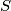$\,S\,$ be a finite set of points in three-dimensional space. Let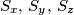$\,S_{x},\,S_{y},\,S_{z}\,$ be the sets consisting of the orthogonal projections of the points of$\,S\,$ onto the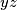$yz$-plane,$zx$-plane,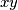$xy$-plane, respectively. Prove that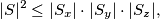where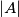$\vert A \vert$ denotes the number of elements in the finite set$A$.

Note Note: The orthogonal projection of a point onto a plane is the foot of the perpendicular from that point to the plane.
Source: Međunarodna matematička olimpijada, shortlist 1992